# Use Thermal Analysis to Predict an IC's Transient Behavior and Avoid Overheating

### Abstract

This article presents a method for predicting thermal behavior in ICs. This information will be especially helpful for the PMICs (power-management ICs) used in automotive applications and other high-temperature environments. After characterizing thermal behavior, we formulate a mathematical model that simulates transient temperatures within the chip. We introduce physical laws governing thermal behavior and evaluate them for use in the thermal-body models defined for an IC. Based on that analysis, we then propose an equivalent passive RC network for modeling an IC's transient thermal behavior. To illustrate an application for the proposed analysis, we devise an RC network for an LED driver (the MAX16828). We conclude with insights on the use and usefulness of this approach, and suggest ways to speed the creation of the RC models.

This article was also featured in Maxim's Engineering Journal, vol. 68 (PDF, 2.72MB).

A similar article appeared in EDN Magazine in January 2010.

Designers often need to know the thermal behavior of an IC, especially for the PMICs (power-management ICs) used in automotive applications. When a particular IC operates at a high temperature (such as +125°C), does it trigger the thermal-shutdown circuitry or exceed the product's safe operating temperature? Without a definite method of analysis, we cannot offer a reliable answer. Therefore, when defining a new IC, we need a way to predict thermal shutdown or excessive die temperature based on complex internal functions.

For operation in DC mode, you can often determine the junction temperature using data-sheet parameters such as θJA (thermal resistance) and θJC (thermal junction temperature).1 However, to predict how high the junction temperature will peak for modes other than DC (such as a power MOSFET driven by a PWM signal to control LEDs or a switching regulator), you need transient thermal data. Although useful, that data is not typically found in data sheets. You might also ask how long the chip can operate at a given power-dissipation level before encountering trouble? That question is also difficult to answer.

This article derives equations that use power dissipation and the ambient temperature to predict the junction temperature of a chip as a function of time. The article begins by introducing the physical laws upon which the analysis is based. The discussion continues by defining an IC system as a complex, layered thermal body. The thermal-body model is then analyzed theoretically, and equations to govern transient thermal behavior are derived. Based on these equations, the article proposes an equivalent RC passive network that represents the IC's thermal characteristics. Finally, to demonstrate the usefulness and accuracy of this analysis, experimental results are shown for a high-voltage, linear HB LED (high-brightness LED) driver with PWM dimming, the MAX16828.

### Laws of Thermal Dynamics

For any object we can derive the required relations for temperature vs. time by using two principal laws.

Newton's law of cooling:(Eq. 1)

Where:
TB is body temperature.
TA is ambient temperature.
kA is a constant of proportionality (> 0).
t is time.

Law of conservation of nonlatent energy: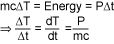(Eq. 2)

Where:
P is constant power generated or imparted to the body.
m is the mass of the body.
c is the specific heat capacity of the body.

Combining these laws, we have:(Eq. 3)

The data sheet for an IC normally lists thermal data for the package, such as θJA. That data lets us analyze the steady-state thermal equilibrium for a package to see if it agrees with Equation 3:at the steady state
Therefore:

 P = mckA(TB - TA) (Eq. 4)

Equation 4 can be rewritten as:(Eq. 5)

Where:
θBA is thermal resistance - body to ambient.
TB is the temperature inside the package.
TA is the ambient temperature outside.

Thus:(Eq. 6)

### Defining the Chip as a Thermal System

A clear definition of the system is very important because the thermal result depends on that definition. In the cross section of a chip mounted on a PCB (Figure 1), we see at least three different materials in the path from die to environment: the die itself, the mold epoxy, and the package. Thermal models are based on one of two patterns of heat flow, depending on the location of the dominant heat source: flow from an external source to the die (when the external source is the dominant heat generator), and flow from the die to the environment (when the die is the dominant heat generator). We will discuss each of these patterns of heat flow in turn.Figure 1. A cross section of a chip mounted on a PCB shows the layers of material between the die and the environment.

### Heat Flow from an External Source to the Chip

Consider the system of Figure 2, which shows a uniform body gaining energy (heat) from a power source and losing energy to the environment.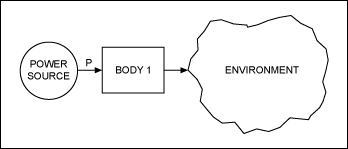Figure 2. This thermal model illustrates the flow of heat from an outside power source to the chip (BODY 1) and then back out to the environment.

Heat reaches the internal die through the package and the mold compound. Therefore, this system also models thermal transients in the chip for heat sources outside the package. The package normally has a much higher thermal resistance than the die itself because the die has lots of metal on it. The die, therefore, tracks the package temperature with almost no lag, thus causing the chip to behave as a single body. We can define this one-body system immediately by using Equation 3. Solving for TB, we have: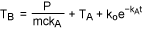(Eq. 7)

Where ko is the constant of integration, which is solved according to the initial conditions. In general, this equation is useful for defining the thermal transient of a chip when the heat source is outside the chip.

We can illustrate this model with an example. To determine the thermal transient for a chip whose initial temperature is Ti, substitute t = 0 and TB = Ti in Equation 7:(Eq. 8)

Therefore: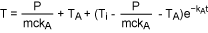(Eq. 9)

Considering the special case for which Ti = TA:(Eq. 10)

Using Equation 6, we can rewrite Equations 9 and 10:(Eq. 11)(Eq. 12)

Equations 11 and 12 are useful for predicting chip temperature (either package or die) when the heat-generating source is outside the package. One example could be a nearby high-current MOSFET that dissipates lots of heat.

When we know kA and θJA we can calculate the temperature at different times. Alternatively, if P is a complex function of time, we can use the above equations to evaluate temperature as a time-based simulation and use MATLAB® software to write a program that plots temperature as a function of time.

The θJA value is provided in data sheets. However, when a setup imposes conditions other than those of the JEDEC standard, that published θJA value for these calculations can cause errors. The JEDEC standard 51-3 states, "It should be emphasized that values measured with these test boards cannot be used to directly predict any particular system application performance, but are for the purposes of comparison between packages."2 Thus, to properly estimate temperature, you should either measure θJA for the prototype board or estimate it directly as explained below.

### Heat Flow from a Die to the Environment

Consider the system of Figure 3, in which a three-body system (similar to a chip) generates heat on the die and dissipates it through the epoxy and package to the environment. Body 1 is the die, Body 2 is the epoxy, and Body 3 is the chip package.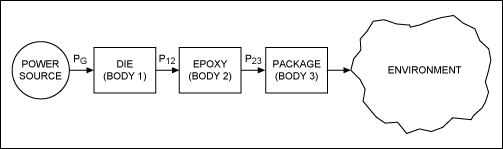Figure 3. Compare this thermal three-body model with the Figure 2 model. Here the flow of heat generated on the die is more complicated.

To solve for θJA in this system, we must define the equations for all three bodies.

Body 1:(Eq. 13)

Body 2:(Eq. 14)

Body 3:(Eq. 15)

Where:
TB1, TB2, TB3 are the instantaneous temperatures of Bodies 1, 2, and 3.
P12 is power in the form of heat transferred from Body 1 to Body 2.
P23 is power in the form of heat transferred from Body 2 to Body 3.
PG is the power generated on Body 1 or directly transferred to Body1.

Power generated by the die (PG) minus power absorbed by the die is:(Eq. 16)

Power received by the epoxy minus power absorbed by the epoxy is: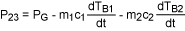(Eq. 17)

Substituting Equations 16 and 17 in Equations 13, 14, and 15: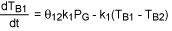(Eq. 18)(Eq. 19)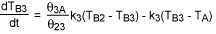(Eq. 20)

The solution of this three-body system in Equations 18, 19, and 20 can be complicated, but the use of Laplace transforms makes it easier. The form of the solution is:
 TB1 = T1em1t + T2em2t + T3em3t + TA + (θ12 + θ23 + θ3A)PG (Eq. 21)

Where:
θ12 is the thermal resistance from Body 1 to Body 2.
θ23 is the thermal resistance from Body 2 to Body 3.
θ3A is the thermal resistance from Body 3 to the environment.
T1, T2, and T3 are the constants of integration.
m1, m2, and m3 are functions of k1, k2, and k3.

Equation 21 predicts die temperature in a very accurate way when the die is generating power. To use this equation, however, we must know all the constants of integration plus m1, m2, and m3, which are complicated functions whose solution is difficult. To avoid this difficult exercise, we use a tool for solving differential equations: SPICE.

### RC Network Models Thermal-Transient Differential Equations

We will now propose a circuit modeled by similar differential equations, and we will then simulate the circuit and read out temperatures from the simulation.

The differential Equations 18, 19, and 20 can be modeled by a simple RC network (Figure 4) that represents the power generated on the die.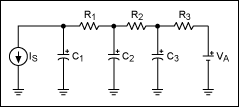Figure 4. This RC network models the transient-thermal behavior of a chip when heat is generated internally.

In Figure 4 initial voltages on the capacitors represent the initial temperatures of the die (C1), the epoxy (C2), and the package (C3). VA represents the ambient temperature of the environment, and IS (the current going into capacitor C1) represents the power generated on the die. The differential equations representing voltages on the capacitors are: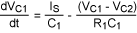(Eq. 22)(Eq. 23)(Eq. 24)

These three equations correspond to Equations 18, 19, and 20, with the following substitutions of variables:

VC1TB1, VC2TB2, VC3TB3, lSPG

The capacitor voltages correspond directly to the temperatures of the die, epoxy, and package. Any SPICE package can simulate the RC circuit easily. When we know the proper values of R1, R2, R3, C1, C2, and C3 modeled for a particular chip, we can then simulate the circuit and directly read out die temperature as the voltage on capacitor C1.

Now we can determine the passive component values for a particular chip (R1, R2, R3, C1, C2, and C3). Use Equation 5 (repeated below as Equation 25) to obtain the thermal resistance for the system (θJA) by measuring the die's steady-state final temperature: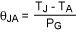(Eq. 25)

Where:
TJ is the steady-state junction temperature of the die.
TA is the ambient temperature.
PG is the power dissipated on the die.

Operating with the same dissipated power (PG) as in Equation 25, you can create a data set for the chip's transient temperature variation by measuring the die temperature at different times starting at time 0. Then, based on the following constraint, do a curve-fitting exercise on the measured data to determine the values of R1, R2, R3, C1, C2, and C3:

 θJA = R1 + R2 + R3 (Eq. 26)

### Measuring the Die Temperature

There are a couple of practical methods to measure the die temperature of an integrated circuit.3 Here we will use the ESD diode forward-drop measurement method to determine the chip temperature, since it is easy and will not introduce a large amount of error. However, to ensure that the accuracy levels of the measurement remain within acceptable limits, always choose the die-temperature measurement technique for a particular chip carefully. The following guidelines will prove helpful.3

1. Make sure that the ESD diode chosen for measurement does not have a large parasitic resistance, nor a large current flow that would offset the diode drop read-out. It is best to discuss this with the IC manufacturer to determine the estimated maximum internal bond-wire and metallization resistance.
2. Also make sure that the ESD diode is near the hotspot of the chip or in the area where you are actually concerned with the temperature. This configuration will provide better estimation of the temperature and deliver more accurate results.
3. If you are choosing a FET's on-resistance as a temperature indicator, make sure that the FET is fully on and in dropout mode at the measurement point.
To use the ESD diode forward-drop approach, we need a diode on the chip to which we can apply forward bias and measure its voltage. That can easily be done on most chips with an ESD diode connected between a pin and the supply voltage. Because the measured data gives us the diode voltage, we must also consider the relationship between a diode voltage and temperature.4

Diode voltage decreases with a nearly constant slope and negligible deviation. If plotted with respect to temperature, the result would look like the plot in Figure 5.Figure 5. The forward voltage for a diode biased at constant current varies with temperature.

In Figure 5, TA is the ambient temperature and VDA is the diode voltage at ambient temperature. We, therefore, know one point on the graph and its slope. Slope can be derived by measuring the diode voltage at different temperatures in a temperature-controlled oven. Alternatively, you can use a number like 2mV/K, which is valid with minimal error for a wide range of diode currents.4 These numbers should apply to any other chip as well, but for accuracy it is always better to measure the slope associated with the current intended for biasing the diode. Any temperature can now be represented in terms of the diode voltage:(Eq. 27)

Where:
T is the temperature for which the diode voltage is VD.
s is the slope of the graph (s < 0).

Substituting this expression in Equations 11 and 12 yields the following:

 VD = sθJAP + VDA + (VDi - sθJAP - VDA)e-kAt (Eq. 28) VD = VDA + sθJAP(1 - e-kAt) (Eq. 29)

Substituting in Equations 18, 19, and 20 also yields:(Eq. 30)(Eq. 31)(Eq. 32)

To apply our RC network properly for curve fitting the measured voltage-transient data for the diode, now we only need to set the magnitude of the current source as:
 lS = sPG (Eq. 33)

Because s < 0, you can realize Equation 33 by reversing the current source direction and setting its magnitude to |sPG|.

### Experimental Determination and Verification of the RC Network

We can demonstrate a practical application of the RC simulation model using the equations derived above and a linear LED driver like the MAX16828/MAX16815. These chips operate up to 40V using few external components, and the MAX16828 supplies an LED string with up to 200mA (Figure 6). The MAX16815 is pin-compatible with the MAX16828 and similar in function, but maximum output current is 100mA instead of 200mA.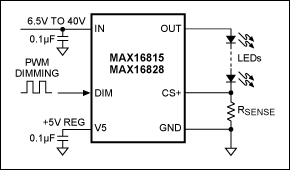Figure 6. Typical application circuit for the MAX16815/MAX16828 HBLED drivers.

Both LED drivers are suitable in automotive applications such as side lighting, automotive exterior rear combination lights, backlighting, and indicators. The MAX16828 can dissipate considerable heat if the internal MOSFET sees high current combined with a large dropout voltage. (The MOSFET does this when the LED string's forward voltage is low.) The voltage across RSENSE is regulated to 200mV ±3.5%, which allows that resistor to set the LED current. The chip's DIM input provides a wide range of PWM dimming for the LEDs and, because it also withstands high voltages, it can connect directly to the IN pin.

To obtain a direct indication of the die temperature, we measure the forward-bias voltage of an internal ESD diode connected between the DIM and IN pins. This diode is biased at ~100µA, causing its forward voltage to vary 2mV/K. (This can be confirmed by heating the part in a temperature-controlled oven.) Figure 7 shows the setup for these experiments. The 5V source and 56kΩ resistor provide 100µA for forward biasing the ESD diode. The driver is programmed to deliver 200mA of output current for the LEDs.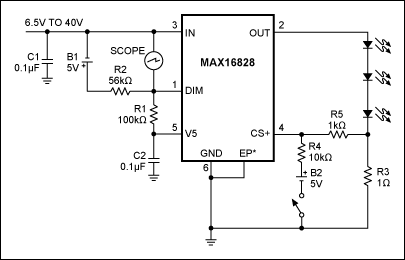Figure 7. The test setup shown lets you measure transient die temperatures using an on-chip ESD diode. *EP indicates an exposed pad.

In this state the part carries a lot of current and our ESD diode measurement is in the path of that measurement. Consequently, we will get some error due to the parasitic resistance of the bond wire and internal metallization. From the internal layout and calculation of the length of bond wire, the worst-case parasitic resistance is estimated to be 50mΩ. With 200mA, this parasitic resistance will cause an error of around ±10mV (max) in our diode reading. Our accuracy error will be larger than ±5°C. Additionally, the ESD diode on the die is placed adjacent to the on-chip power MOSFET device and thermal-shutdown circuitry. This configuration makes the diode the best indicator of that region's temperature.

### System Definition 1

This next section describes how you can use a test setup to capture transient-thermal diode voltages for use in the system-definition equations presented above in Equations 7 and 21.

To calculate kA and θJA (for substitution in Equation 11), we heat the chip using a hot-air gun. The chip should be powered off because we do not want to generate internal heat. Heating the part with a hot-air gun causes the temperature of the package and die to rise together. You can monitor the die's temperature change by measuring the diode voltage on a scope (Figure 8).Figure 8. This diode-voltage transient includes exponential curves that represent heating with an external heat gun (falling curve) and cooling by removal of the heat gun (rising curve).

When the chip is heated, the diode voltage decreases with an exponential rate of change as the equation predicts. Near the center of the curve the hot-air gun is switched off, causing the package and die to begin cooling. The diode voltage rises, again following an exponential curve.

We do not know exactly how much heat is imparted from the heat gun to the chip. Therefore, to eliminate that unknown we first adjust Equation 28 to fit only the rising (cooling) part of the curve (Figure 8). This curve-fitting exercise lets us estimate the best value for kA. With no heat power transferred to the package during cooling, the package is simply cooling down with P = 0. Equation 28, therefore, simplifies to:

 VDB = VDA + (VDi - VDA)e-kAt (Eq. 34)

We know the values for VDA (643mV from the initial measurement at room temperature) and VDi (the reading for t = 0 reference). To determine kA, we must just adjust the equation so that it includes a couple of readings on the rising curve. This exercise yields kA = -0.0175. A graph of the readings (diode voltages in mV, with respect to time in seconds) and Equation 34 with the above kA is shown in Figure 9.Figure 9. Equation 34, fitted to a couple of diode-voltage measurements, closely tracks all the diode measurements for a chip that is cooling after being heated with a heat gun.

As we can see in Figure 9, Equation 34 closely follows the measured data for kA = -0.0175. To verify that our equations are correct, we try to fit the falling curve on Equation 28 with the value determined for kA. The equation fits very accurately (Figure 10). Thus, we see that Equation 34 for the system discussed in System definition 1 closely matches the experimental data.Figure 10. The curve-fitted Equation 28 closely tracks diode-voltage measurements for the falling (heating) portion of the curve.

### System Definition 2

Verification of the system 2 Equations 30, 31, and 32 is more difficult. We must generate heat on the die, measure the die temperature using the diode forward voltage, and fit that temperature value to a simulated value for the C1 voltage of the proposed RC network. This task is accomplished by writing a program using MATLAB.

It is important to record the thermal transient at a time for which the initial temperature of the whole chip is known. In that way we also know the initial capacitor voltages for solving the RC network. Using the same test setup (see Figure 7), we now turn on the current and capture the diode voltage on a scope (Figure 11).Figure 11. A forward-voltage transient from the MAX16828's internal diode signals that an on-board MOSFET has turned on and is generating heat.

Similar transients are recorded for three different power-dissipation levels, and one curve is fitted to that data. The circuit in Figure 12 is the result of fitting from the first set of data in which the power dissipation is 1.626W. The graph in Figure 13 compares the measured and fitted data. Similarly, the graph in Figure 14 shows how the RC network fits the second set of readings (power dissipation of 2.02W). The graph in Figure 15 shows how it fits the third set of readings (power dissipation of 1.223W).Figure 12. With component values as shown, this RC network models the chip's thermal transient when heat is generated on the die.Figure 13. Measured vs. curve-fitted results for the chip's heating curve when the die is dissipating 1.626W.Figure 14. Measured vs. curve-fitted results for the chip's heating curve when the die is dissipating 2.02W.Figure 15. Measured vs. curve-fitted results for the chip's heating curve when the die is dissipating 1.223W.

These experimental results show that the measured results closely match the theoretical model. Such modeling is useful for simulating the transient temperature of an IC, once you have modeled the RC network for that particular chip. The model can also be used for chips of similar size to determine their thermal characteristics during the definition phase. That capability can indicate the operational limitations of the chip. That information, in turn, helps you define the chip's operational modes to prevent overheating.

### Conclusion

This article has described a way to model the thermal behavior of a chip as an RC network, which can then be simulated easily using a SPICE tool. The following measures can improve the accuracy of this model:

• Take data at both extremes of power dissipation and at one level in the middle. Fitting the RC network to all three levels simultaneously makes the model usable for most practical power-dissipation levels.
• Improve model accuracy by collecting data points at different ambient temperatures.
These exercises should improve accuracy when necessary, but most applications do not require that you know the temperature with high accuracy. Applications and design engineers, as well as system designers may find the method useful. For more detailed chip information, a company can create RC network models for its ICs and make them available with the chips' corresponding SPICE models.

References

1. "Package Thermal Resistance Values (Theta JA and Theta JC) for Dallas Semiconductor Temperature Sensors," Maxim application note 3930.
2. "Package Thermal Characteristics," Actel Corporation application note AC220, (February 2005).
3. Rako, Paul, "Hot, cold, and broken: Thermal-design techniques," EDN online (3/29/2007).
4. Pease, Bob, National Semiconductor, "The Best of Bob Pease. What's All This VBE Stuff, Anyhow?" (11/5/2008).
*Da Weng is no longer with Maxim.